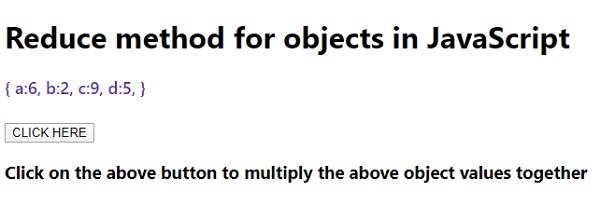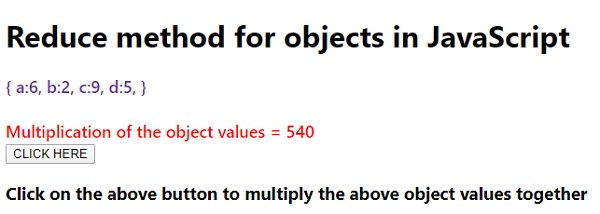# How to apply the reduce method for objects in JavaScript?

Following is the code to apply reduce method to objects in JavaScript −

## Example

Live Demo

<!DOCTYPE html>
<html lang="en">
<meta charset="UTF-8" />
<meta name="viewport" content="width=device-width, initial-scale=1.0" />
<title>Document</title>
<style>
body {
font-family: "Segoe UI", Tahoma, Geneva, Verdana, sans-serif;
}
.result,.sample {
font-size: 18px;
font-weight: 500;
color: rebeccapurple;
}
.result {
color: red;
}
</style>
<body>
<h1>Reduce method for objects in JavaScript</h1>
<div class="sample">{ a:6, b:2, c:9, d:5, }</div>
<div class="result"><br /></div>
<h3>Click on the above button to multiply the above object values together</h3>
<script>
let resEle = document.querySelector(".result");
let BtnEle = document.querySelector(".Btn");
let multiplyObj = {
a: 6,
b: 2,
c: 9,
d: 5,
};
let multiplyTotal = Object.values(multiplyObj).reduce((a, b) => a * b);
resEle.innerHTML += "Multiplication of the object values = " + multiplyTotal;
});
</script>
</body>
</html>

## Output

The above code will produce the following output −